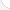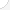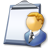Medical records and health information techniciansDescription: These workers organize and keep track of patients' medical records. First, they make sure all the right forms have been signed. Then they put the information into a computer file. Finally, they code the information so that it can be pulled up easily. They may work day, evening, or night shifts. Health information technicians must be computer literate and pay attention to details.Source: Bureau of Labor Statistics, U.S. Department of Labor, Occupational Outlook Handbook, 2008-09 Edition at http://www.bls.gov/OCO/Complete Job Profile: http://www.bls.gov/oco/ocos103.htmSalary: \$23,001 to \$28,000 per yearComments:

There are 50 math topics Medical records and health information technicians need to know.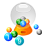Basic Math / Algebra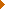FractionsDecimalsRatio and ProportionPercentCustomary MeasurementMetric MeasurementMeasurement ConversionBasic ProbabilityBasic StatisticsStatistical GraphingPowers and RootsNegative NumbersBasic Problem Solving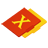First-Year AlgebraUsing FormulasLinear EquationsLinear InequalitiesOperations with PolynomialsFactoring PolynomialsRational ExpressionsCoordinate Graphing 2DLinear SystemsQuadratic EquationsAlgebraic Representation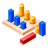GeometryBasic TerminologyAngle MeasurementSimilarityGeometric MeanRight Triangle TrigonometryCirclesAreaVolumeMake/Use 3D Drawings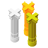Second-Year Algebra / TrigonometryFunctionsVariationLogarithmsAdvanced ProbabilityAdvanced StatisticsConic SectionsNon-Linear SystemsTrigonometric/Circular FunctionsGraphs of Trigonometric FunctionsVectors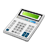Other TopicsBasic Calculator UseScientific Calculator UseComputer UseGroup Problem SolvingMental MathInductive/Deductive ReasoningMath CommunicationsMathematical Modeling